21

# Acids and Bases

Acid-base reactions involve the transfer of hydrogen ions between reactants. A compound that can donate a proton (a hydrogen ion) to another compound is called a Brønsted-Lowry acid. The compound that accepts the proton is called a Brønsted-Lowry base. The species remaining after a Brønsted-Lowry acid has lost a proton is the conjugate base of the acid. The species formed when a Brønsted-Lowry base gains a proton is the conjugate acid of the base. Thus, an acid-base reaction occurs when a proton is transferred from an acid to a base, with formation of the conjugate base of the reactant acid and formation of the conjugate acid of the reactant base.

## 21.1 Acid-Base Reactions

An acid-base reaction is one in which a hydrogen ion, H+, is transferred from one chemical species to another. Such reactions are of central importance to numerous natural and technological processes, ranging from the chemical transformations that take place within cells and the lakes and oceans, to the industrial-scale production of fertilizers, pharmaceuticals, and other substances essential to society. The subject of acid-base chemistry, therefore, is worthy of thorough discussion, and a full chapter is devoted to this topic later in the text.

For purposes of this brief introduction, we will consider only the more common types of acid-base reactions that take place in aqueous solutions. In this context, an acid is a substance that will dissolve in water to yield hydronium ions, H3O+. As an example, consider the equation shown here:

$\text{HCl}\left(aq\right)+{\text{H}}_{2}\text{O}\left(aq\right)\phantom{\rule{0.2em}{0ex}}⟶\phantom{\rule{0.2em}{0ex}}{\text{Cl}}^{\text{−}}\left(aq\right)+{\text{H}}_{3}{\text{O}}^{\text{+}}\left(aq\right)$

The process represented by this equation confirms that hydrogen chloride is an acid. When dissolved in water, H3O+ ions are produced by a chemical reaction in which H+ ions are transferred from HCl molecules to H2O molecules (Figure 22.1).

Figure 21.1

When hydrogen chloride gas dissolves in water, (a) it reacts as an acid, transferring protons to water molecules to yield (b) hydronium ions (and solvated chloride ions).

The nature of HCl is such that its reaction with water as just described is essentially 100% efficient: Virtually every HCl molecule that dissolves in water will undergo this reaction. Acids that completely react in this fashion are called strong acids, and HCl is one among just a handful of common acid compounds that are classified as strong (Table 21.1). A far greater number of compounds behave as weak acids and only partially react with water, leaving a large majority of dissolved molecules in their original form and generating a relatively small amount of hydronium ions. Weak acids are commonly encountered in nature, being the substances partly responsible for the tangy taste of citrus fruits, the stinging sensation of insect bites, and the unpleasant smells associated with body odor. A familiar example of a weak acid is acetic acid, the main ingredient in food vinegars:

${\text{CH}}_{3}{\text{CO}}_{2}\text{H}\left(aq\right)+{\text{H}}_{2}\text{O}\left(l\right)⇌{\text{CH}}_{3}{\text{CO}}_{2}{}^{\text{−}}\left(aq\right)+{\text{H}}_{3}{\text{O}}^{\text{+}}\left(aq\right)$

When dissolved in water under typical conditions, only about 1% of acetic acid molecules are present in the ionized form, ${\text{CH}}_{3}{\text{CO}}_{2}{}^{-}$ (Figure 21.2). (The use of a double-arrow in the equation above denotes the partial reaction aspect of this process, a concept addressed fully in the chapters on chemical equilibrium.)

Figure 21.2

(a) Fruits such as oranges, lemons, and grapefruit contain the weak acid citric acid. (b) Vinegars contain the weak acid acetic acid. (credit a: modification of work by Scott Bauer; credit b: modification of work by Brücke-Osteuropa/Wikimedia Commons)

Table 21.3

Common Strong Acids

Compound FormulaName in Aqueous Solution
HBrhydrobromic acid
HClhydrochloric acid
HIhydroiodic acid
HNO3nitric acid
HClO4perchloric acid
H2SO4sulfuric acid

A base is a substance that will dissolve in water to yield hydroxide ions, OH. The most common bases are ionic compounds composed of alkali or alkaline earth metal cations (groups 1 and 2) combined with the hydroxide ion—for example, NaOH and Ca(OH)2. Unlike the acid compounds discussed previously, these compounds do not react chemically with water; instead they dissolve and dissociate, releasing hydroxide ions directly into the solution. For example, KOH and Ba(OH)2 dissolve in water and dissociate completely to produce cations (K+ and Ba2+, respectively) and hydroxide ions, OH. These bases, along with other hydroxides that completely dissociate in water, are considered strong bases.

Consider as an example the dissolution of lye (sodium hydroxide) in water:

$\text{NaOH}\left(s\right)\phantom{\rule{0.2em}{0ex}}⟶\phantom{\rule{0.2em}{0ex}}{\text{Na}}^{\text{+}}\left(aq\right)+{\text{OH}}^{\text{−}}\left(aq\right)$

This equation confirms that sodium hydroxide is a base. When dissolved in water, NaOH dissociates to yield Na+ and OH ions. This is also true for any other ionic compound containing hydroxide ions. Since the dissociation process is essentially complete when ionic compounds dissolve in water under typical conditions, NaOH and other ionic hydroxides are all classified as strong bases.

Unlike ionic hydroxides, some compounds produce hydroxide ions when dissolved by chemically reacting with water molecules. In all cases, these compounds react only partially and so are classified as weak bases. These types of compounds are also abundant in nature and important commodities in various technologies. For example, global production of the weak base ammonia is typically well over 100 metric tons annually, being widely used as an agricultural fertilizer, a raw material for chemical synthesis of other compounds, and an active ingredient in household cleaners (Figure 21.3). When dissolved in water, ammonia reacts partially to yield hydroxide ions, as shown here:

${\text{NH}}_{3}\left(aq\right)+{\text{H}}_{2}\text{O}\left(l\right)⇌{\text{NH}}_{4}{}^{\text{+}}\left(aq\right)+{\text{OH}}^{\text{−}}\left(aq\right)$

This is, by definition, an acid-base reaction, in this case involving the transfer of H+ ions from water molecules to ammonia molecules. Under typical conditions, only about 1% of the dissolved ammonia is present as ${\text{NH}}_{4}{}^{+}$ ions.

Figure 21.4

Ammonia is a weak base used in a variety of applications. (a) Pure ammonia is commonly applied as an agricultural fertilizer. (b) Dilute solutions of ammonia are effective household cleansers. (credit a: modification of work by National Resources Conservation Service; credit b: modification of work by pat00139)

A neutralization reaction is a specific type of acid-base reaction in which the reactants are an acid and a base (but not water), and the products are often a salt and water

$\text{acid}+\text{base}\phantom{\rule{0.2em}{0ex}}⟶\phantom{\rule{0.2em}{0ex}}\text{salt}+\text{water}$

To illustrate a neutralization reaction, consider what happens when a typical antacid such as milk of magnesia (an aqueous suspension of solid Mg(OH)2) is ingested to ease symptoms associated with excess stomach acid (HCl):

$\text{Mg}{\text{(OH)}}_{2}\left(s\right)+2\text{HCl}\left(aq\right)\phantom{\rule{0.2em}{0ex}}⟶\phantom{\rule{0.2em}{0ex}}{\text{MgCl}}_{2}\left(aq\right)+2{\text{H}}_{2}\text{O}\left(l\text{).}$

Note that in addition to water, this reaction produces a salt, magnesium chloride.

### EXAMPLE 21.1.1

#### Writing Equations for Acid-Base Reactions

Write balanced chemical equations for the acid-base reactions described here:

(a) the weak acid hydrogen hypochlorite reacts with water

(b) a solution of barium hydroxide is neutralized with a solution of nitric acid

#### Solution

(a) The two reactants are provided, HOCl and H2O. Since the substance is reported to be an acid, its reaction with water will involve the transfer of H+ from HOCl to H2O to generate hydronium ions, H3O+ and hypochlorite ions, OCl.
$\text{HOCl}\left(aq\right)+{\text{H}}_{2}\text{O}\left(l\right)⇌{\text{OCl}}^{\text{−}}\left(aq\right)+{\text{H}}_{3}{\text{O}}^{\text{+}}\left(aq\right)$

A double-arrow is appropriate in this equation because it indicates the HOCl is a weak acid that has not reacted completely.

(b) The two reactants are provided, Ba(OH)2 and HNO3. Since this is a neutralization reaction, the two products will be water and a salt composed of the cation of the ionic hydroxide (Ba2+) and the anion generated when the acid transfers its hydrogen ion $\left({\text{NO}}_{3}{}^{\text{−}}\right).$

$\text{Ba}{\text{(OH)}}_{2}\left(aq\right)+2{\text{HNO}}_{3}\left(aq\right)\phantom{\rule{0.2em}{0ex}}⟶\phantom{\rule{0.2em}{0ex}}\text{Ba}\left({\text{NO}}_{3}\right){}_{2}\left(aq\right)+2{\text{H}}_{2}\text{O}\left(l\right)$

Write the net ionic equation representing the neutralization of any strong acid with an ionic hydroxide. (Hint: Consider the ions produced when a strong acid is dissolved in water.)

${\text{H}}_{3}{\text{O}}^{\text{+}}\left(aq\right)+{\text{OH}}^{\text{−}}\left(aq\right)\phantom{\rule{0.2em}{0ex}}⟶\phantom{\rule{0.2em}{0ex}}2{\text{H}}_{2}\text{O}\left(l\right)$

### 21.1.2 CHEMISTRY IN EVERYDAY LIFE

#### Stomach Antacids

Our stomachs contain a solution of roughly 0.03 M HCl, which helps us digest the food we eat. The burning sensation associated with heartburn is a result of the acid of the stomach leaking through the muscular valve at the top of the stomach into the lower reaches of the esophagus. The lining of the esophagus is not protected from the corrosive effects of stomach acid the way the lining of the stomach is, and the results can be very painful. When we have heartburn, it feels better if we reduce the excess acid in the esophagus by taking an antacid. As you may have guessed, antacids are bases. One of the most common antacids is calcium carbonate, CaCO3. The reaction,

${\text{CaCO}}_{3}\left(s\right)+2\text{HCl}\left(aq\right)\phantom{\rule{0.2em}{0ex}}⇌\phantom{\rule{0.2em}{0ex}}{\text{CaCl}}_{2}\left(aq\right)+{\text{H}}_{2}\text{O}\left(l\right)+{\text{CO}}_{2}\left(g\right)$

not only neutralizes stomach acid, it also produces CO2(g), which may result in a satisfying belch.

Milk of Magnesia is a suspension of the sparingly soluble base magnesium hydroxide, Mg(OH)2. It works according to the reaction:

$\text{Mg}{\left(\text{OH}\right)}_{2}\left(s\right)\phantom{\rule{0.2em}{0ex}}⇌\phantom{\rule{0.2em}{0ex}}{\text{Mg}}^{2+}\left(aq\right)+2{\text{OH}}^{\text{−}}\left(aq\right)$

The hydroxide ions generated in this equilibrium then go on to react with the hydronium ions from the stomach acid, so that:

${\text{H}}_{3}{\text{O}}^{\text{+}}+{\text{OH}}^{\text{−}}\phantom{\rule{0.2em}{0ex}}⇌\phantom{\rule{0.2em}{0ex}}2{\text{H}}_{2}\text{O}\left(l\right)$

This reaction does not produce carbon dioxide, but magnesium-containing antacids can have a laxative effect. Several antacids have aluminum hydroxide, Al(OH)3, as an active ingredient. The aluminum hydroxide tends to cause constipation, and some antacids use aluminum hydroxide in concert with magnesium hydroxide to balance the side effects of the two substances.

### 21.1.3 CHEMISTRY IN EVERYDAY LIFE

#### Culinary Aspects of Chemistry

Examples of acid-base chemistry are abundant in the culinary world. One example is the use of baking soda, or sodium bicarbonate in baking. NaHCO3 is a base. When it reacts with an acid such as lemon juice, buttermilk, or sour cream in a batter, bubbles of carbon dioxide gas are formed from decomposition of the resulting carbonic acid, and the batter “rises.” Baking powder is a combination of sodium bicarbonate, and one or more acid salts that react when the two chemicals come in contact with water in the batter.

Many people like to put lemon juice or vinegar, both of which are acids, on cooked fish (Figure 21.4). It turns out that fish have volatile amines (bases) in their systems, which are neutralized by the acids to yield involatile ammonium salts. This reduces the odor of the fish, and also adds a “sour” taste that we seem to enjoy.

Figure 21.5

A neutralization reaction takes place between citric acid in lemons or acetic acid in vinegar, and the bases in the flesh of fish.

Pickling is a method used to preserve vegetables using a naturally produced acidic environment. The vegetable, such as a cucumber, is placed in a sealed jar submerged in a brine solution. The brine solution favors the growth of beneficial bacteria and suppresses the growth of harmful bacteria. The beneficial bacteria feed on starches in the cucumber and produce lactic acid as a waste product in a process called fermentation. The lactic acid eventually increases the acidity of the brine to a level that kills any harmful bacteria, which require a basic environment. Without the harmful bacteria consuming the cucumbers they are able to last much longer than if they were unprotected. A byproduct of the pickling process changes the flavor of the vegetables with the acid making them taste sour.

## 21.2 Brønsted-Lowry Acids and Bases

### Learning Objectives

By the end of this section, you will be able to:

• Identify acids, bases, and conjugate acid-base pairs according to the Brønsted-Lowry definition
• Write equations for acid and base ionization reactions
• Use the ion-product constant for water to calculate hydronium and hydroxide ion concentrations
• Describe the acid-base behavior of amphiprotic substances

The acid-base reaction class has been studied for quite some time. In 1680, Robert Boyle reported traits of acid solutions that included their ability to dissolve many substances, to change the colors of certain natural dyes, and to lose these traits after coming in contact with alkali (base) solutions. In the eighteenth century, it was recognized that acids have a sour taste, react with limestone to liberate a gaseous substance (now known to be CO2), and interact with alkalis to form neutral substances. In 1815, Humphry Davy contributed greatly to the development of the modern acid-base concept by demonstrating that hydrogen is the essential constituent of acids. Around that same time, Joseph Louis Gay-Lussac concluded that acids are substances that can neutralize bases and that these two classes of substances can be defined only in terms of each other. The significance of hydrogen was reemphasized in 1884 when Svante Arrhenius defined an acid as a compound that dissolves in water to yield hydrogen cations (now recognized to be hydronium ions) and a base as a compound that dissolves in water to yield hydroxide anions.

Johannes Brønsted and Thomas Lowry proposed a more general description in 1923 in which acids and bases were defined in terms of the transfer of hydrogen ions, H+. (Note that these hydrogen ions are often referred to simply as protons, since that subatomic particle is the only component of cations derived from the most abundant hydrogen isotope, 1H.) A compound that donates a proton to another compound is called a Brønsted-Lowry acid, and a compound that accepts a proton is called a Brønsted-Lowry base. An acid-base reaction is, thus, the transfer of a proton from a donor (acid) to an acceptor (base).

The concept of conjugate pairs is useful in describing Brønsted-Lowry acid-base reactions (and other reversible reactions, as well). When an acid donates H+, the species that remains is called the conjugate base of the acid because it reacts as a proton acceptor in the reverse reaction. Likewise, when a base accepts H+, it is converted to its conjugate acid. The reaction between water and ammonia illustrates this idea. In the forward direction, water acts as an acid by donating a proton to ammonia and subsequently becoming a hydroxide ion, OH, the conjugate base of water. The ammonia acts as a base in accepting this proton, becoming an ammonium ion, ${{\text{NH}}_{4}}^{\text{+}}\text{,}$ the conjugate acid of ammonia. In the reverse direction, a hydroxide ion acts as a base in accepting a proton from ammonium ion, which acts as an acid.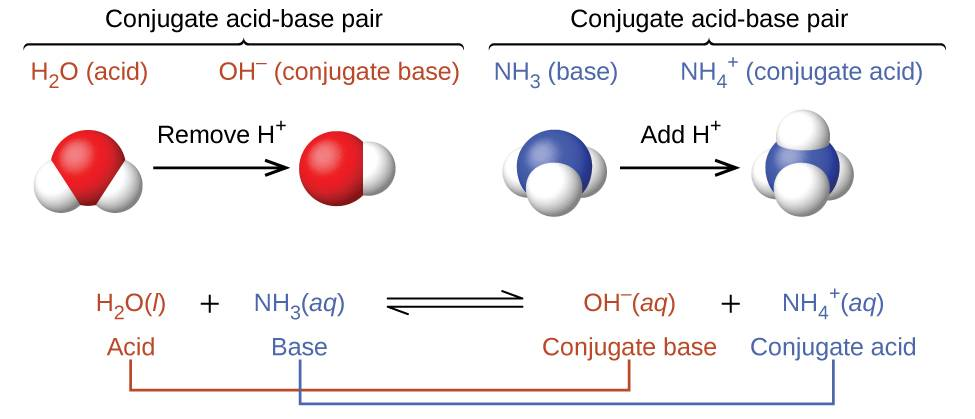The reaction between a Brønsted-Lowry acid and water is called acid ionization. For example, when hydrogen fluoride dissolves in water and ionizes, protons are transferred from hydrogen fluoride molecules to water molecules, yielding hydronium ions and fluoride ions: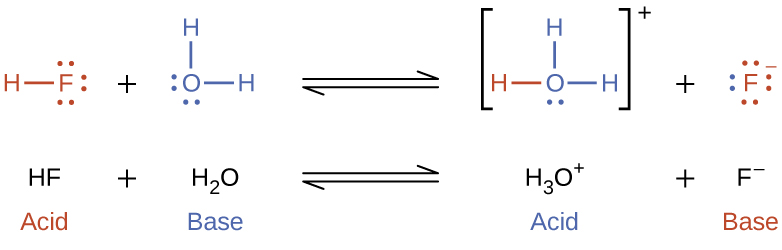Base ionization of a species occurs when it accepts protons from water molecules. In the example below, pyridine molecules, C5NH5, undergo base ionization when dissolved in water, yielding hydroxide and pyridinium ions: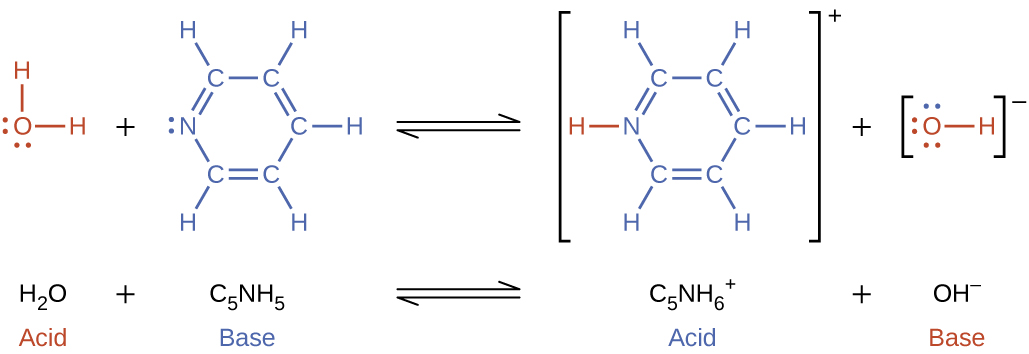The preceding ionization reactions suggest that water may function as both a base (as in its reaction with hydrogen fluoride) and an acid (as in its reaction with ammonia). Species capable of either donating or accepting protons are called amphiprotic, or more generally, amphoteric, a term that may be used for acids and bases per definitions other than the Brønsted-Lowry one. The equations below show the two possible acid-base reactions for two amphiprotic species, bicarbonate ion and water:

${\text{HCO}}_{3}{}^{–}\left(\text{aq}\right)+{\text{H}}_{2}\text{O}\left(\text{l}\right)\phantom{\rule{4em}{0ex}}{\text{CO}}_{3}{}^{2–}\left(\text{aq}\right)+{\text{H}}_{3}{\text{O}}^{+}\left(\text{aq}\right)$
${\text{HCO}}_{3}{}^{–}\left(\text{aq}\right)+{\text{H}}_{2}\text{O}\left(\text{l}\right)\phantom{\rule{4em}{0ex}}{\text{H}}_{2}{\text{CO}}_{3}\left(\text{aq}\right)+{\text{OH}}^{–}\left(\text{aq}\right)$

The first equation represents the reaction of bicarbonate as an acid with water as a base, whereas the second represents reaction of bicarbonate as a base with water as an acid. When bicarbonate is added to water, both these equilibria are established simultaneously and the composition of the resulting solution may be determined through appropriate equilibrium calculations, as described later in this chapter.

In the liquid state, molecules of an amphiprotic substance can react with one another as illustrated for water in the equations below: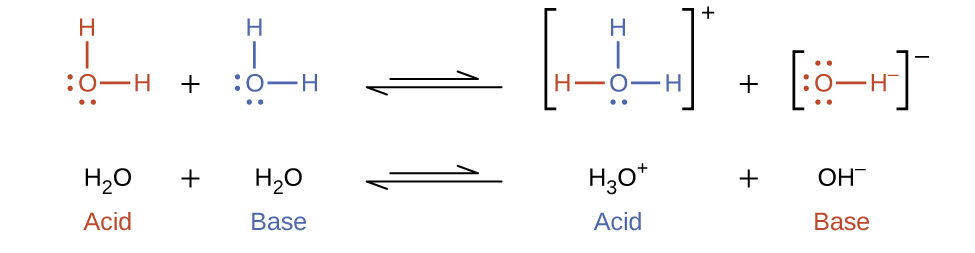### EXAMPLE 21.2.1

#### Ion Concentrations in Pure Water

What are the hydronium ion concentration and the hydroxide ion concentration in pure water at 25 °C?

#### Solution

The autoionization of water yields the same number of hydronium and hydroxide ions. Therefore, in pure water, [H3O+] = [OH] = x. At 25 °C:
${K}_{\text{w}}=\left[{\text{H}}_{3}{\text{O}}^{\text{+}}\right]\left[{\text{OH}}^{\text{−}}\right]=\left(x\right)\left(x\right)={x}^{2}=1.0\phantom{\rule{0.2em}{0ex}}×\phantom{\rule{0.2em}{0ex}}{10}^{-14}$

So:

$x=\left[{\text{H}}_{3}{\text{O}}^{\text{+}}\right]=\left[{\text{OH}}^{\text{−}}\right]=\sqrt{1.0\phantom{\rule{0.2em}{0ex}}×\phantom{\rule{0.2em}{0ex}}{10}^{-14}}=1.0\phantom{\rule{0.2em}{0ex}}×\phantom{\rule{0.2em}{0ex}}{10}^{-7}\phantom{\rule{0.2em}{0ex}}M$

The hydronium ion concentration and the hydroxide ion concentration are the same, 1.0 $×$ 10−7 M.

The ion product of water at 80 °C is 2.4 $×$ 10−13. What are the concentrations of hydronium and hydroxide ions in pure water at 80 °C?

[H3O+] = [OH] = 4.9 $×$ 10−7 M

### EXAMPLE 21.2.2

#### Representing the Acid-Base Behavior of an Amphoteric Substance

Write separate equations representing the reaction of ${\text{HSO}}_{3}{}^{\text{−}}$

(a) as an acid with OH

(b) as a base with HI

#### Solution

(a) ${\text{HSO}}_{3}{}^{\text{−}}\left(aq\right)+{\text{OH}}^{\text{−}}\left(aq\right)\phantom{\rule{0.2em}{0ex}}⇌\phantom{\rule{0.2em}{0ex}}{\text{SO}}_{3}{}^{\text{2−}}\left(aq\right)+{\text{H}}_{2}\text{O}\left(l\right)$

(b) ${\text{HSO}}_{3}{}^{\text{−}}\left(aq\right)+\text{HI}\left(aq\right)\phantom{\rule{0.2em}{0ex}}⇌\phantom{\rule{0.2em}{0ex}}{\text{H}}_{2}{\text{SO}}_{3}\left(aq\right)+{\text{I}}^{\text{−}}\left(aq\right)$

Write separate equations representing the reaction of ${\text{H}}_{2}{\text{PO}}_{4}{}^{\text{−}}$

(a) as a base with HBr

(b) as an acid with OH

(a) ${\text{H}}_{2}{\text{PO}}_{4}{}^{\text{−}}\left(aq\right)+\text{HBr}\left(aq\right)\phantom{\rule{0.2em}{0ex}}⇌\phantom{\rule{0.2em}{0ex}}{\text{H}}_{3}{\text{PO}}_{4}\left(aq\right)+{\text{Br}}^{\text{−}}\left(aq\right);$ (b) ${\text{H}}_{2}{\text{PO}}_{4}{}^{\text{−}}\left(aq\right)+{\text{OH}}^{\text{−}}\left(aq\right)\phantom{\rule{0.2em}{0ex}}⇌\phantom{\rule{0.2em}{0ex}}{\text{HPO}}_{4}{}^{\text{2−}}\left(aq\right)+{\text{H}}_{2}\text{O}\left(l\right)$

## 21.3 Effect of Molecular Structure on Acid-Base Strength

#### Binary Acids and Bases

In the absence of any leveling effect, the acid strength of binary compounds of hydrogen with nonmetals (A) increases as the H-A bond strength decreases down a group in the periodic table. For group 17, the order of increasing acidity is HF < HCl < HBr < HI. Likewise, for group 16, the order of increasing acid strength is H2O < H2S < H2Se < H2Te.

Across a row in the periodic table, the acid strength of binary hydrogen compounds increases with increasing electronegativity of the nonmetal atom because the polarity of the H-A bond increases. Thus, the order of increasing acidity (for removal of one proton) across the second row is CH4 < NH3 < H2O < HF; across the third row, it is SiH4 < PH3 < H2S < HCl (see Figure 21.5).

Figure 21.6

The figure shows trends in the strengths of binary acids and bases.

## 21.4 Ternary Acids and Bases

Ternary compounds composed of hydrogen, oxygen, and some third element (“E”) may be structured as depicted in the image below. In these compounds, the central E atom is bonded to one or more O atoms, and at least one of the O atoms is also bonded to an H atom, corresponding to the general molecular formula OmE(OH)n. These compounds may be acidic, basic, or amphoteric depending on the properties of the central E atom. Examples of such compounds include sulfuric acid, O2S(OH)2, sulfurous acid, OS(OH)2, nitric acid, O2NOH, perchloric acid, O3ClOH, aluminum hydroxide, Al(OH)3, calcium hydroxide, Ca(OH)2, and potassium hydroxide, KOH: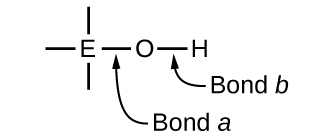If the central atom, E, has a low electronegativity, its attraction for electrons is low. Little tendency exists for the central atom to form a strong covalent bond with the oxygen atom, and bond a between the element and oxygen is more readily broken than bond b between oxygen and hydrogen. Hence bond a is ionic, hydroxide ions are released to the solution, and the material behaves as a base—this is the case with Ca(OH)2 and KOH. Lower electronegativity is characteristic of the more metallic elements; hence, the metallic elements form ionic hydroxides that are by definition basic compounds.

If, on the other hand, the atom E has a relatively high electronegativity, it strongly attracts the electrons it shares with the oxygen atom, making bond a relatively strongly covalent. The oxygen-hydrogen bond, bond b, is thereby weakened because electrons are displaced toward E. Bond b is polar and readily releases hydrogen ions to the solution, so the material behaves as an acid. High electronegativities are characteristic of the more nonmetallic elements. Thus, nonmetallic elements form covalent compounds containing acidic −OH groups that are called oxyacids.

Increasing the oxidation number of the central atom E also increases the acidity of an oxyacid because this increases the attraction of E for the electrons it shares with oxygen and thereby weakens the O-H bond. Sulfuric acid, H2SO4, or O2S(OH)2 (with a sulfur oxidation number of +6), is more acidic than sulfurous acid, H2SO3, or OS(OH)2 (with a sulfur oxidation number of +4). Likewise nitric acid, HNO3, or O2NOH (N oxidation number = +5), is more acidic than nitrous acid, HNO2, or ONOH (N oxidation number = +3). In each of these pairs, the oxidation number of the central atom is larger for the stronger acid (Figure 21.6).

Figure 21.7

As the oxidation number of the central atom E increases, the acidity also increases.

Hydroxy compounds of elements with intermediate electronegativities and relatively high oxidation numbers (for example, elements near the diagonal line separating the metals from the nonmetals in the periodic table) are usually amphoteric. This means that the hydroxy compounds act as acids when they react with strong bases and as bases when they react with strong acids. The amphoterism of aluminum hydroxide, which commonly exists as the hydrate Al(H2O)3(OH)3, is reflected in its solubility in both strong acids and strong bases. In strong bases, the relatively insoluble hydrated aluminum hydroxide, Al(H2O)3(OH)3, is converted into the soluble ion, ${\left[\text{Al}{\left({\text{H}}_{2}\text{O}\right)}_{2}\left({\text{OH}\right)}_{4}\right]}^{\text{−}},$ by reaction with hydroxide ion:

$\text{Al}{\left({\text{H}}_{2}\text{O}\right)}_{3}\left({\text{OH}\right)}_{3}\left(aq\right)+{\text{OH}}^{\text{−}}\left(aq\right)\phantom{\rule{0.2em}{0ex}}⇌\phantom{\rule{0.2em}{0ex}}{\text{H}}_{2}\text{O}\left(l\right)+{\left[\text{Al}{\left({\text{H}}_{2}\text{O}\right)}_{2}\left({\text{OH}\right)}_{4}\right]}^{\text{−}}\left(aq\right)$

In this reaction, a proton is transferred from one of the aluminum-bound H2O molecules to a hydroxide ion in solution. The Al(H2O)3(OH)3 compound thus acts as an acid under these conditions. On the other hand, when dissolved in strong acids, it is converted to the soluble ion ${\left[\text{Al}{\left({\text{H}}_{2}\text{O}\right)}_{6}\right]}^{3+}$ by reaction with hydronium ion:

${\text{3H}}_{3}{\text{O}}^{\text{+}}\left(aq\right)+\text{Al}{\left({\text{H}}_{2}\text{O}\right)}_{3}\left({\text{OH}\right)}_{3}\left(aq\right)\phantom{\rule{0.2em}{0ex}}⇌\phantom{\rule{0.2em}{0ex}}\text{Al}{\left({\text{H}}_{2}\text{O}\right)}_{6}{}^{3+}\left(aq\right)+{\text{3H}}_{2}\text{O}\left(l\right)$

In this case, protons are transferred from hydronium ions in solution to Al(H2O)3(OH)3, and the compound functions as a base.

Previous Citation(s)
Flowers, P., et al. (2019). Chemistry: Atoms First 2e. https://openstax.org/details/books/chemistry-atoms-first-2e (7.2, 14.1, 14.3)